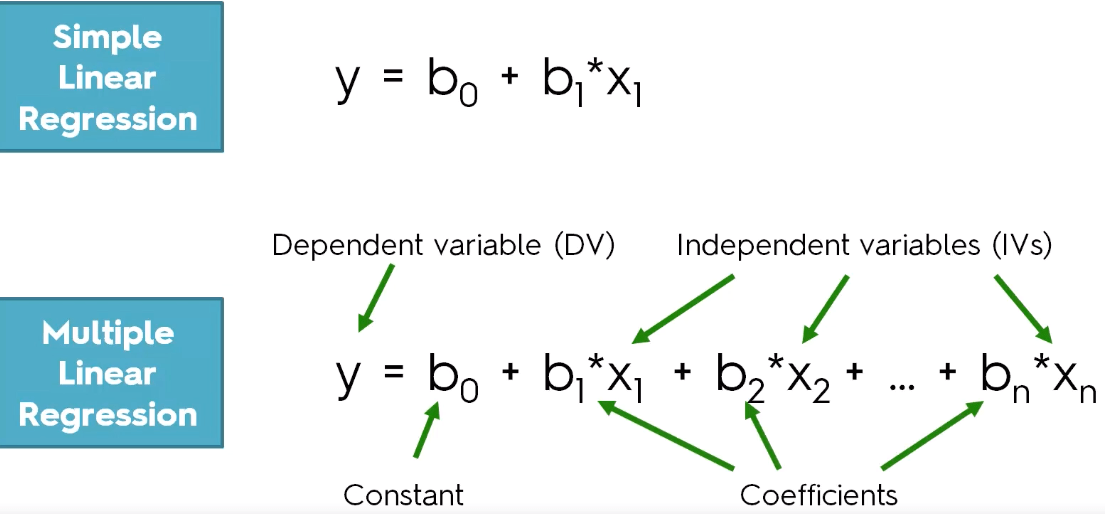December 11, 2023Disclaimer: Lottery games are based on random chance and it is not possible to predict the outcome of a draw using any type of mathematical or statistical model. Predicting a random event is virtually impossible. I am not making and promises nor guarantees that this actually works. This is all for fun!

Draft Notice: If this notice is still here, that means it’s a draft. I’m leaving this draft open just so that search engines could pick this page up in search results. Please come back periodically for updated content.

Introduction:

Now that we have the disclaimer out of the way, I would first like to explain why I decided to write a framework for a Python Script that can possibly predict the winning numbers for MegaMillions Lottery. The answer is simple. Wishful thinking and pure curiosity. I am not a mathematician but I do dabble a bit in math since it’s part of my necessary skills as an engineer or a coder. I had to do a bit of research on various Mathematical Algorithms to get this script to almost work. Specifically statistics and probability mathematics. But first, what is an algorithm? Algorithm in simple terms is a process or sets of steps or a formula to be followed to complete a calculation. Specifically, a mathematical calculation.

The algorithm I used in this project is called Linear Regression. Linear Regression model is a common model or a set of formulas and calculations that is used in finance to predict various financial models such as stock prices. More precisely, linear regression is used to determine the character and strength of the association between a dependent variable and a series of other independent variables. It helps create models to make predictions, such as predicting a company’s stock price (G2.COM 2017).
Linear Regression is also used to predict trends and make forecasts for various data types.

I. Introduction

• Definition of linear regression
• Overview of the linear regression model algorithm
• Importance of linear regression in data analysis

II. Types of linear regression

• Simple linear regression
• Multiple linear regression

III. Implementing linear regression in Python

• Required libraries (e.g. numpy, pandas, scikit-learn)
• Data preparation and exploration
• Model training and evaluation
• Fine-tuning model hyperparameters

IV. Applications of linear regression in Python

• Predicting continuous target variables for MegaMillion winning numbers
• Analyzing the impact of multiple predictor variables on a target variable
• Comparing the effectiveness of different regression models on a given dataset

V. Advantages and limitations of linear regression

• Advantages (e.g. simplicity, interpretability, fast training and prediction times)
• Limitations (e.g. assumption of linear relationship, sensitivity to outliers)

VI. Conclusion

• Recap of key points covered in the paper
• Future directions for further exploration of linear regression in Python